JEE  >  Irodov Solutions: Molecules and Crystals- 3

# Irodov Solutions: Molecules and Crystals- 3 - JEE

Q.201. Calculate the interval (in eV units) between neighbouring levels of free electrons in a metal at T = 0 near the Fermi level, if the concentration of free electrons is n = 2.0.1022  cm-3  and the volume of the metal is V = 1.0 cm3

Ans. We write the expression for the number of electrons as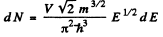Hence if A £ is the spacing between neighbouring levels near the Fermi level we must have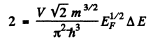(2 on the RHS is to take care of both spins / electrons). Thus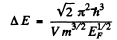But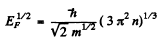So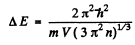Substituting the data we get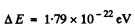Q.202. Making use of Eq. (6.4g), find at T = 0:
(a) the velocity distribution of free electrons;
(b) the ratio of the mean velocity of free electrons to their maximum velocity.

Ans. (a) From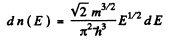we get on using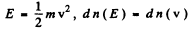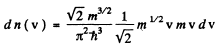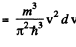This holds for 0 < v < vF where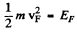and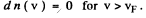(b) Mean velocity is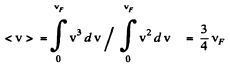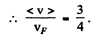Q.203. On the basis of Eq. (6.4g) find the number of free electrons in a metal at T = 0 as a function of de Broglie wavelengths

Ans. Using the formula of the previous section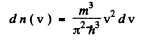We put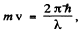where λ = de Broglie wavelength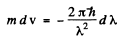Taking account of the fact that λ decreases when v increases we write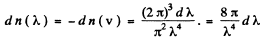Q.204. Calculate the electronic gas pressure in metallic sodium, at T = 0, in which the concentration of free electrons is n = = 2.5.1022 cm-3. Use the equation for the pressure of ideal gas.

Ans. From the kinetic theory of gasses we know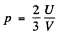Here U is the total interval energy of the gas. This result is applicable to Fermi gas also Now at T = 0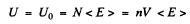so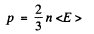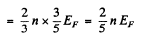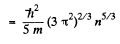Substituting the values we get

p = 4.92 x 104 atmos

Q.205. The increase in temperature of a cathode in electronic tube by ΔT = 1.0 K from the value T= 2000 K results in the increase of saturation current by η =  1.4%. Find the work function of electron for the material of the cathode.

Ans. From Richardson's equation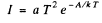where A is the work function in eV. When T increases by ΔT , I increases to (1 + η)I. Then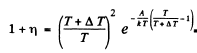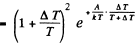Expanding and neglecting higher powers of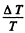we get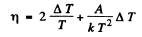Thus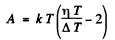Substituting we get            A = 4.48 eV

Q.206. Find the refractive index of metallic sodium for electrons with kinetic energy T = 135 eV. Only one free electron is assumed to correspond to each sodium atom.

Ans.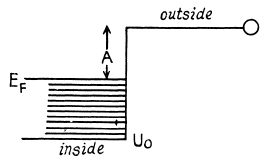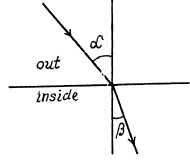The potential energy inside the metal is - U0 for the electron and it related to the work function A by

U0 = Ep + A

If T is the K.E. of electrons outside the metal, its K.E. inside the metal will be (E + U0) . On entering the metal electron connot experience any tangential force so the tangential component of momentum is unchanged. Then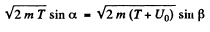Hence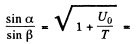n by the definition of refractive index

In sodium with one free electron per Na atom

n = 2.54 x 1022 per c.c.

EF = 3.15 eV

A = 2.27 eV (from table)

U0 = 5.42 eV

n = 102

Q.207. Find the minimum energy of electron-hole pair formation in an impurity-free semiconductor whose electric conductance increases η = 5.0 times when the temperature increases from T1  = = 300 K to T2 = 400 K.

Ans. In a pure (intrinsic) semiconductor the conductivity is related to the temperature by the following formula very closely :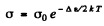where Δε is the eneigy gap between the top of valence band and the bottom of conduction band; it is also the minimum energy required for the formation of electron-hole pair. The conductivity increases with temperature and we have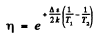or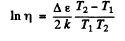Hence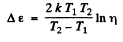Substitution gives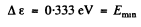Q.208. At very low temperatures the photoelectric threshold short wavelength in an impurity-free germanium is equal to λih = 1.7μm. Find the temperature coefficient of resistance of this germanium sample at room temperature.

Ans. The photoelectric threshold determines the band gap Δε by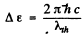On the other hand the temperature coefficient of resistance is defined by (p is resistivity)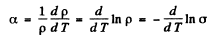where σ is the conductivity. But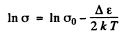Then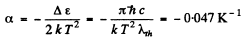Q.209. Fig. 6.11 illustrates logarithmic electric conductance as a function of reciprocal temperature (7' in kK units) for some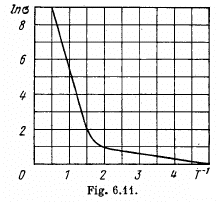n-type semiconductor. Using this plot, find the width of the forbidden band of the semiconductor and the activation energy of donor levels.

Ans. At high temperatures (small values of T-1) most of the conductivity is intrinsic i.e. it is due to the transition of electrons from the upper levels of the valance band into the lower levels of conduction vands.
For this we can apply approximately the formulaor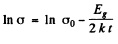From this we get the band gap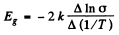The slope must be calculated at small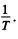Evaluation gives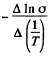= 7000 K

Hence              Eg = 1.21 eV

At low temperatures (high values of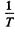) the conductance is mostly due to impurities. If E0 is the ionization energy of donor levels then we can write the approximate formula (valid at low temperature)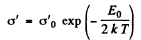So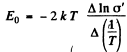The slope must be calculated at low temperatures. Evaluation gives the slope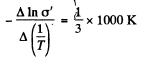Then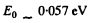Q.210. The resistivity of an impurity-free semiconductor at room temperature is ρ = 50 Ω•cm. It becomes equal to ρ= 40 Ω•cm when the semiconductor is illuminated with light, and t = 8 ms after switching off the light source the resistivity becomes equal to ρ2 = 45 Ω•cm. Find the mean lifetime of conduction electrons and holes.

Ans. We write the conductivity of the sample as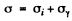where σi = intrinsic conductivity and σγ is the photo conductivity. At t = 0, assuming saturation we have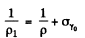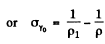Time t after light source is switched off

we have because of recombination of electron and holes in the sample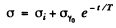where T = mean lifetime of electrons and holes.

Thus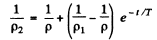or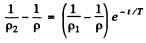or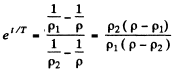Hence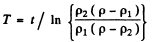Substitution gives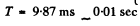Q.211. In Hall effect measurements a plate of width h = 10 mm and length l = 50 mm made of p-type semiconductor was placed in a magnetic field with induction B = 5.0 kG. A potential difference V = 10 V was applied across the edges of the plate. In this case the Hall field is V= 50 mV and resistivity ρ = 2.5 Ω•cm. Find the concentration of holes and hole mobility.

Ans.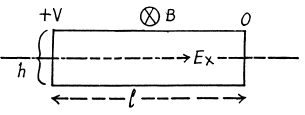We shall ignore minority carriers.
Drifting holes experience a sideways force in the magnetic field and react by setting up a Hall electric field Eγ to counterbalance it Thus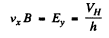If the concentration of carriers is n then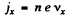Hence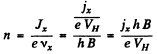Also using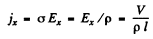we get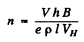Substituting the data (note that in MKS units B = 5.0 k G = 0.5 T)

ρ = 2.5 x 10-2 ohm-m

we get        n = 4.99 x 1021 m -3

= 4.99 x 1015 per cm3

Also the mobility is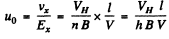Substitution gives  U= 0.05 m2/ V - s

Q.212. In Hall effect measurements in a magnetic field with induction B = 5.0 kG the transverse electric field strength in an impurity-free germanium turned out to be η = 10 times less than the longitudinal electric field strength. Find the difference in the mobilities of conduction electrons and holes in the given semiconductor.

Ans.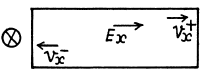If an electric Geld Ex is present in a sample containing equal amounts of both electrons and holes, the two drift in opposite directions.
In the presence of a magnetic field Bz = B they set up Hall voltages in opposite directions.

The net Hall electric field is given by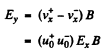But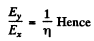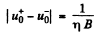Substitution gives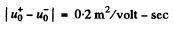Q.213. The Hall effect turned out to be not observable in a semiconductor whose conduction electron mobility was η = 2.0 times that of the hole mobility. Find the ratio of hole and conduction electron concentrations in that semiconductor

Ans.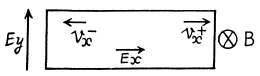When the sample contains unequal number of carriers of both types whose mobilities are different, static equilibrium (i.e. no transverse movement of either electron or holes) is impossible in a magnetic Geld. The transverse electric Geld acts differently on electrons and holes. If the Ey that is set up is as shown, the net Lorentz force per unit charge (effective transverse electric Geld) on electrons is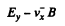and on holes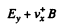(we are assuming B = Bz). There is then a transverse drift of electrons and holes and the net transverse current must vanish in equilibrium. Using mobility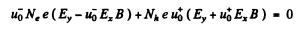or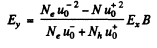On the other hand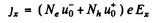Thus, the Hall coefficient is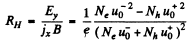We see that RH = 0 when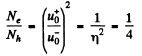The document Irodov Solutions: Molecules and Crystals- 3 - JEE is a part of JEE category.
All you need of JEE at this link: JEE
Signup to see your scores go up within 7 days! Learn & Practice with 1000+ FREE Notes, Videos & Tests.
10M+ students study on EduRev
Track your progress, build streaks, highlight & save important lessons and more!(Scan QR code)
Related Searches

,

,

,

,

,

,

,

,

,

,

,

,

,

,

,

,

,

,

,

,

,

;